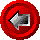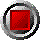Level-coupon European & American Bond Options (HW-Tree)Level-coupon European & American Bond Options (HW-Tree)

HWTree_BondAmerCall(argument list…)

HWTree_BondAmerPut(argument list…)

HWTree_BondEuroCall(argument list…)

HWTree_BondEuroPut(argument list…)

These functions return the values of American and European call and put options on coupon-bearing bonds using the Hull-White trinomial tree interest rate model. The functions use the following arguments:

 Argument Description Restrictions Valuation_Date valuation date (e.g. today) valid Excel date number Bond_Settlement_Lag number of business days until settlement for bond transaction made on Valuation_Date >= 0 Bond_Maturity_Date bond maturity date > Valuation_Date (with settlement lag) Coupon annual bond coupon in decimal form (e.g. six percent entered as 0.06) >= 0 Freq number of bond coupons per annum 1, 2, 4, or 12 DCB day count basis 0 = 30/360 (US) 1 = act/act for CAD/US T-Bonds 2 = act/360 3 = act/365 4 = 30/360 (European) Option_Maturity option expiry date valid Excel date number >= Valuation_Date <= Bond_Maturity_Date Strike option strike per \$100 par > 0 Zero_Dates array of zero coupon curve dates strictly ascending order The first date of this array must be Valuation_Date Zero_Rates array of continuously compounded riskless rates in decimal form corresponding to Zero_Dates > 0 Short_Rate_Vol annual standard deviation of the short rate of interest, in decimal form > 0 Reversion_Rate mean reversion rate of the short rate of interest, in decimal form >= 0 OAS parallel shift of the zero curve in decimal form Bucket_Start start of bucket for zero curve shifts set to 0 if curve shift is not desired Bucket_End end of bucket for zero curve shift >= Bucket_Start Bucket_Shift parallel shift of the zero curve between Bucket_Start and Bucket_End in decimal form set to 0 if curve shock is not desired Tree_Steps number of steps in trinomial tree > 0, typically 25-50 Holidays array of holiday dates valid Excel date numbers strictly ascending order

© 1995-98 Leap of Faith Research Inc.